# Chapter 14 Graphing Networks

## 14.1 Vertices and edges

A network is a set of connections between objects. A network combines information about two different kinds of things:

1. The objects themselves, generally called vertices Vertices: The objects that are being connected in a network. Sometimes called “nodes”.
2. The connections — called edges Edge: The connections between pairs of vertices in a network – between pairs of vertices.

Figure 14.1: A network of relationships between the genes expressed in cancer cells from different people.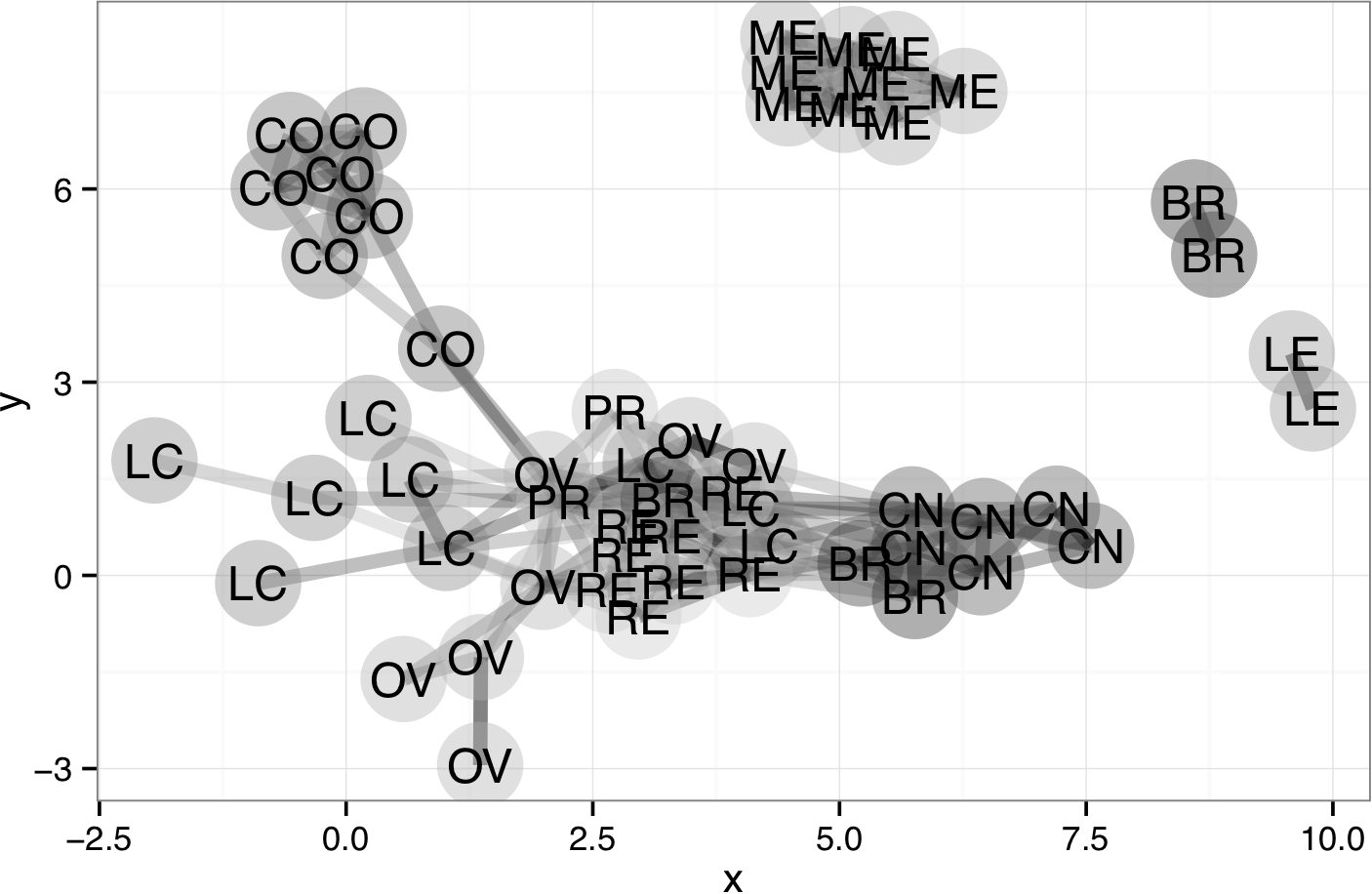In the previous examples in this book, the end-result of data wrangling has been a single, glyph-ready data frame. With networks, things are different. A network always involves two different kinds of things — vertices and edges. Accordingly, representing a network with data always involves two different data frames:

• A data frame for the vertices, giving the properties of each vertex. In Figure 14.1 the vertices are samples of cancer cells and are shown as large dots with colors and labels identifying the type of cancer. A data frame representing the vertices might look like Table 14.1.

Table 14.1: A data frame representing the vertices in Figure 14.1.

ID cancerType
TK_10 renal
MDA_MB_35 melanoma
MCF7 breast
… and so on for 60 rows altogether.
• A different data frame for the edges. Each case in this table has at least two attributes: the origin vertex of the edge and the destination vertex of the edge. The data frame might look like Table 14.2.

Table 14.2: A data frame representing the edges in Figure 14.1.

origin dest
MCF7 LOXIMV1
MCF7 UO_31
LOXIMVI HL_60
SK_MEL_5 NCI_H226
SK_OV_3 LOXIMV1
HL_60 TK_10
… and so on for 241 rows altogether.

The following sections show some of the ways that data wrangling can be used in determining what are the edges and what determines the location of each vertex in the graphic.

## 14.2 Example: Migration Networks

The `MigrationFlows` data frame presents how many people migrate between one country and another. Table 14.3 shows `Bilateral`, a wrangled form of `MigrationFlows` listing how many women migrated from one country to another and vice versa.

Table 14.3: `Bilateral`, which shows emigration and immigration as different variables.

CountryA CountryB BtoA AtoB
POL DEU 2961 1112903
PAK IND 2923092 3995350
ITA USA 1394 599174
DEU USA 7838 572383
GBR CAN 32687 487980
IND LKA 15290 465760
… and so on for 53,592 rows altogether.

`Bilateral` describes edges: the links between countries. In a data frame describing edges, the case will be an edge. The minimal information needed to describe an edge is the identities of the two vertices connected by the edge.

To describe the vertices, a different data frame is required, one where each case is one vertex. The vertex data frame can contain other information about each vertex. For countries, that information might be the population or land area or GPD of each country. The glyph-ready vertex data frame should also describe where each vertex is to be located on the graph. `CountryCentroids` is a vertex data frame, giving the latitude and longitude of each country.

Table 14.4: CountryCentroids

name iso_a3 long lat
Afghanistan AFG 66.168500 33.78231
Aland ALA 19.967041 60.19722
Albania ALB 20.259880 41.14326
Algeria DZA 2.828547 28.14225
… and so on for 241 rows altogether.

To show the vertices graphically, the information in the vertex data frame can be used to generate a scatter plot like Figure 14.2.

Figure 14.2: Locations of the vertices described by `CountryCentroids`. Another layer shows country boundaries.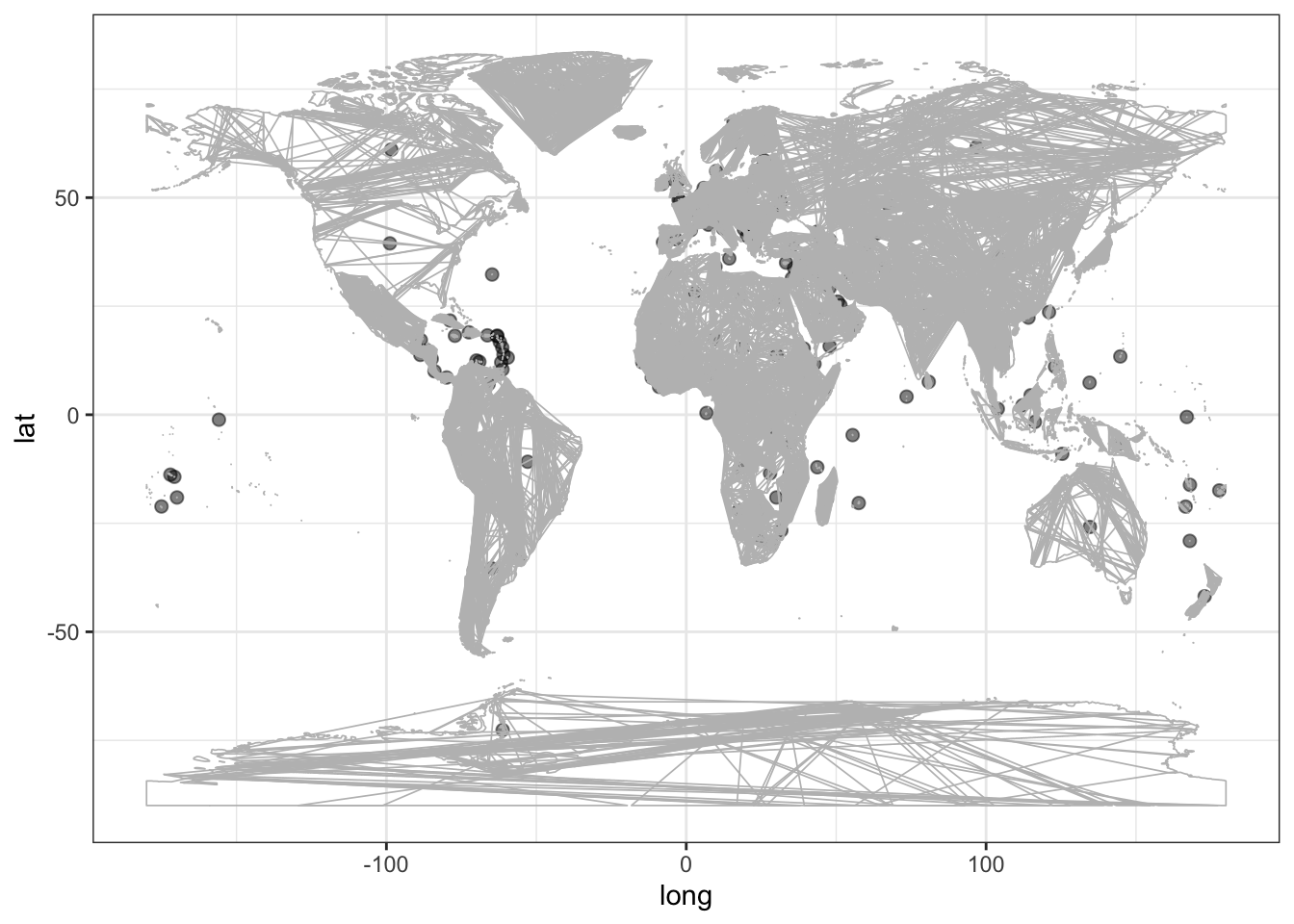`Bilateral` lists the edges, which can be drawn as line segments. But `Bilateral` is not glyph-ready for drawing the edges. Drawing an edge requires four location attributes: x and y of the vertex at one end of the edge, and x and y of the vertex at the other end. The location of each vertex is contained in the vertex data frame, `CountryCentroids`. In order to wrangle `Bilateral` into a glyph-ready form, the location information from `CountryCentroids` is joined to it. This requires two join steps: joining the location of the vertex for `countryA` and similarly joining the location of the vertex for `countryB`. The result is the `Edges` data frame (Table 14.5).

Table 14.5: `Edges`, a data frame based on `Bilateral` wrangled into a form that is glyph-ready for drawing network edges.

CountryA CountryB BtoA AtoB longA latA longB latB
FRA DZA 201387 78867 2.72 46.53 2.83 28.14
FRA AUS 8385 1925 2.72 46.53 134.61 -25.85
FRA AUT 7100 7460 2.72 46.53 14.32 47.60
FRA BEN 114 142 2.72 46.53 2.55 9.62
FRA AND 111 111 2.72 46.53 1.53 42.53
FRA AGO 3140 684 2.72 46.53 17.74 -12.36
… and so on for 1,672 rows altogether.

Drawing the edges can be done in the usual way. The “segment” geom is an appropriate glyph:

``````Edges %>%
ggplot(aes(x = longA, y = latA, xend = longB, yend = latB)) +
geom_segment(alpha = 0.2)``````

Figure 14.3: The edges in `Bilateral` using latitude and longitude to mark the location of each vertex.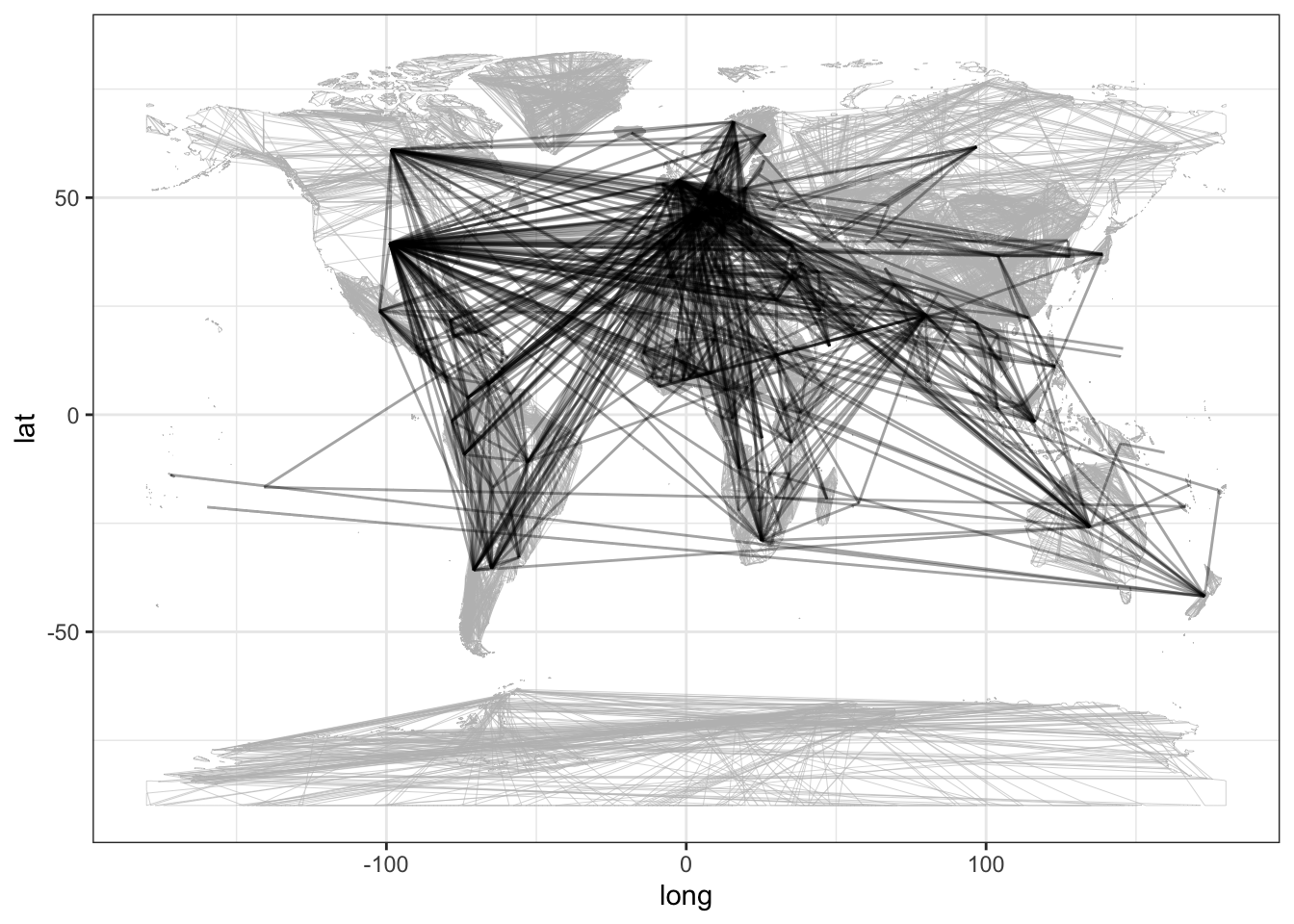## 14.3 Vertex Location from the Edges

It’s perfectly sensible to show the migration network using latitude and longitude for the vertex location. But many networks, for instance the gene expression network in Figure 14.1, have vertices with no natural location. Indeed, even when there is a natural location for each vertex, that might not be the best way to draw the network. Looking at Figure 14.3 critically, you can see that the graphic is dominated by the country pairs that are far apart, e.g. China and the US, Japan and Brazil, the UK and Australia. Migration among European countries, on the other hand, is compressed into a small part of the graphic. There are many crossings where the person viewing the graph can get confused.

Sometimes displays of networks are better when the vertices are placed to put them close to the vertices to which they are linked. Instead of identifying an attribute of the vertices, the location is set by the edges in a way that minimizes crossings or by some other criterion.

It’s challenging to figure out the best places for vertices to minimize the edge crossings and to keep connected vertices together. Several different methods have been described in the network-drawing literature. To simplify things, the `DataComputing` packageThe `igraph` package provides the power behind the location algorithms. provides two functions, `edgesToVertices()` and `edgesForPlotting()` to do this automatically.

To illustrate, consider a network to display cultural affinity between countries. As a simplistic measure of “cultural affinity,” use the balance between inbound and outbound migration between two countries and the number of people migrating. Here’s one way:

``````CulturalAffinity <-
Bilateral %>%
mutate(balance = pmin(AtoB / BtoA, BtoA / AtoB))``````

`CulturalAffinity` contains information about edges, but it has an edge even for country pairs with unbalanced migration. To show strong cultural affinity, eliminate the country pairs with unbalanced or small migration.

``````BigAffinity <-
CulturalAffinity %>%
filter(balance > 0.5, AtoB > 500 | BtoA > 500)``````

There are 316 edges in `BigAffinity`. Use `edgesToVertices()` to position the vertices in a way that minimizes edge crossing.

``````Vertices <-
BigAffinity %>%
edgesToVertices(from = CountryA, to = CountryB)
Vertices``````

Table 14.6: Vertex location derived from `CulturalAffinity` using `edgesToVertices()`.

ID x y
FRA -2.2706798 1.745457
GHA 1.6835417 4.868318
HKG 0.4330789 3.914866
ISL -5.6353426 5.071588
AFG -1.4635977 -5.377435
DZA -1.8672736 -1.541071
… and so on for 125 rows altogether.

Now that the vertex location has been determined, that location can be joined with the edge data in `CulturalAffinity`. This will enable the edges to be drawn connecting the vertices.

``````Edges <-
Vertices %>%
edgesForPlotting(ID = ID, x, y,
Edges = BigAffinity,
from = CountryA, to = CountryB )

Edges %>%
arrange(desc(balance))``````
CountryB CountryA BtoA AtoB balance x y xend yend
DEU ITA 34794 34918 0.9964488 -3.128883 -0.3618877 -4.293726 -1.1476551
ITA DEU 34918 34794 0.9964488 -4.293726 -1.1476551 -3.128883 -0.3618877
DEU IND 513 516 0.9941860 -3.044984 -3.0998401 -4.293726 -1.1476551
IND DEU 516 513 0.9941860 -4.293726 -1.1476551 -3.044984 -3.0998401
CUB JAM 2366 2352 0.9940828 10.770438 5.5470831 10.600987 6.2576916
JAM CUB 2352 2366 0.9940828 10.600987 6.2576916 10.770438 5.5470831
… and so on for 316 rows altogether.

Finally, a plot of the network:

``````Edges %>%
ggplot(aes(x = x, y = y)) +
geom_segment(aes(xend = xend, yend = yend, alpha = balance)) +
geom_point(data = Vertices, size = 5, color = "white", aes(x = x, y = y)) +
geom_text(data = Vertices, size = 3, aes(x = x, y = y, label=ID)) +
theme(panel.background = element_blank(), legend.position="top")``````

Figure 14.4: Links between countries with balanced two-way migration.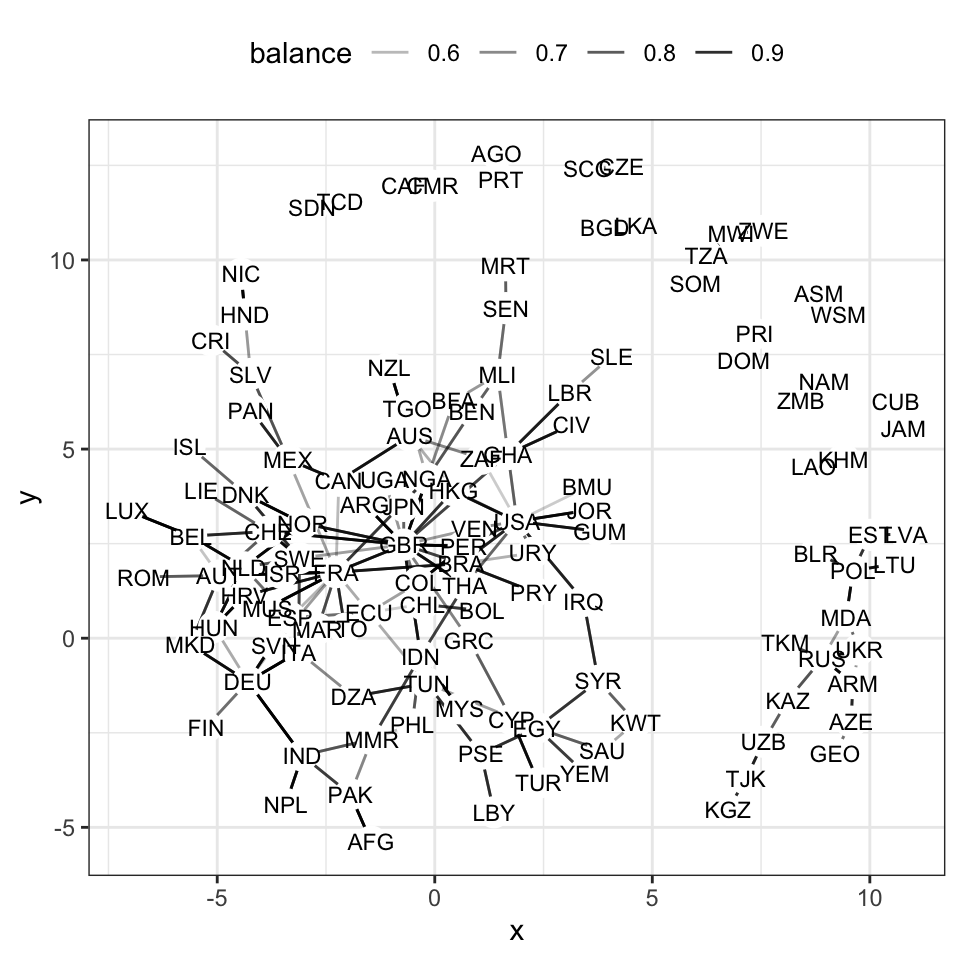Even though the countries are not presented by geographic location, the network helps to illustrate the patterns of affinity between countries. Great Britain (GBR), the USA, and France (FRA) each are hubs having affinities with several countries. Egypt (EGY) is the center of an Arabic network connecting Saudi Arabia, Yemen, Kuwait, Palestine, Syria, and Tunisia. Another cluster centered on Russia (RUS) connects countries formed after the break-up of the Soviet Union.

## 14.4 Exercises

Problem 14.1: Here is a network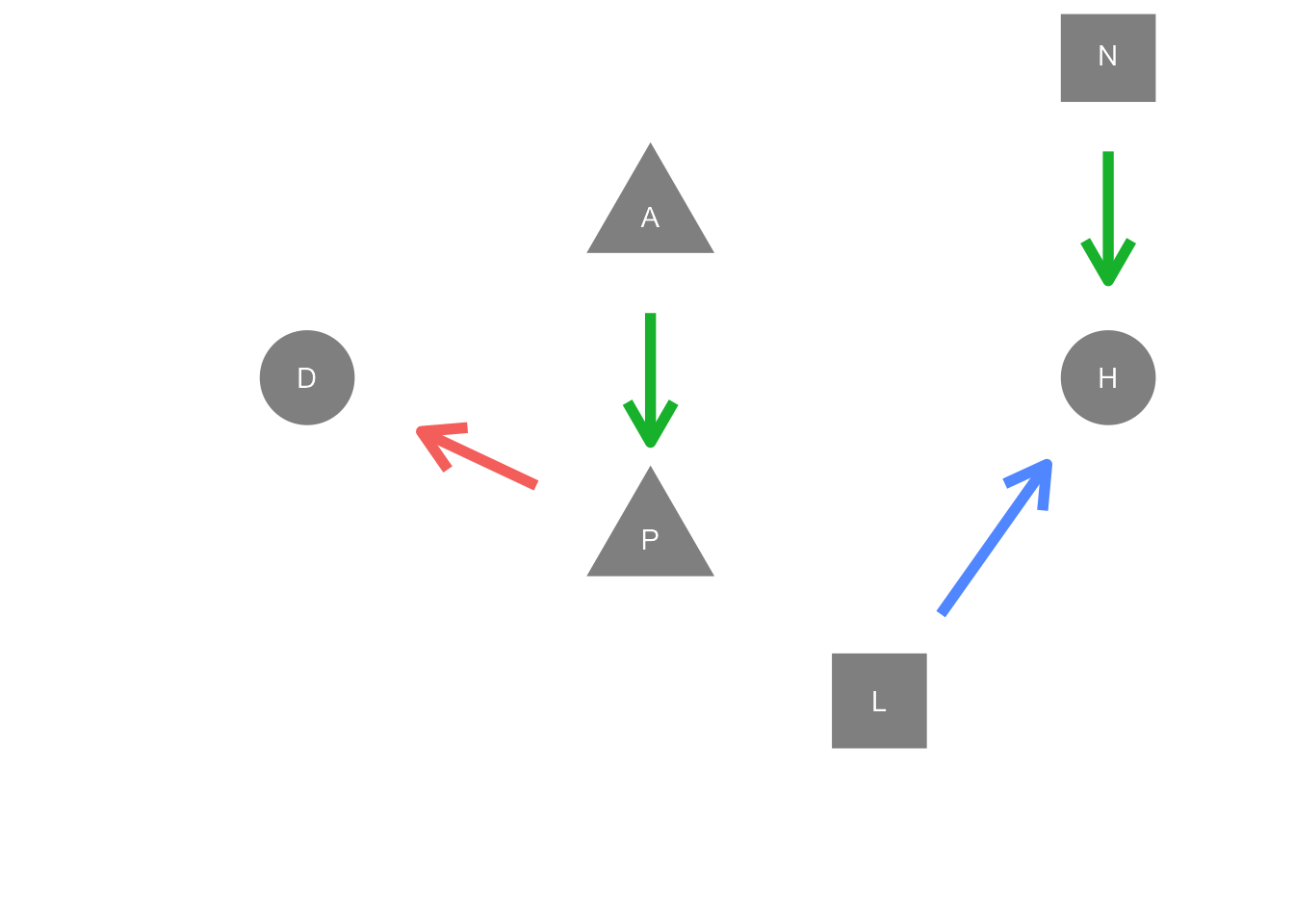Which table gives the information needed to draw the edges in the graph?

Table 1        Table 2        Table 3
A -> P A -> P P -> A
H -> D P -> D P -> N
L -> H L -> H H -> L
A -> N N -> H N -> H

Problem 14.2: For this network …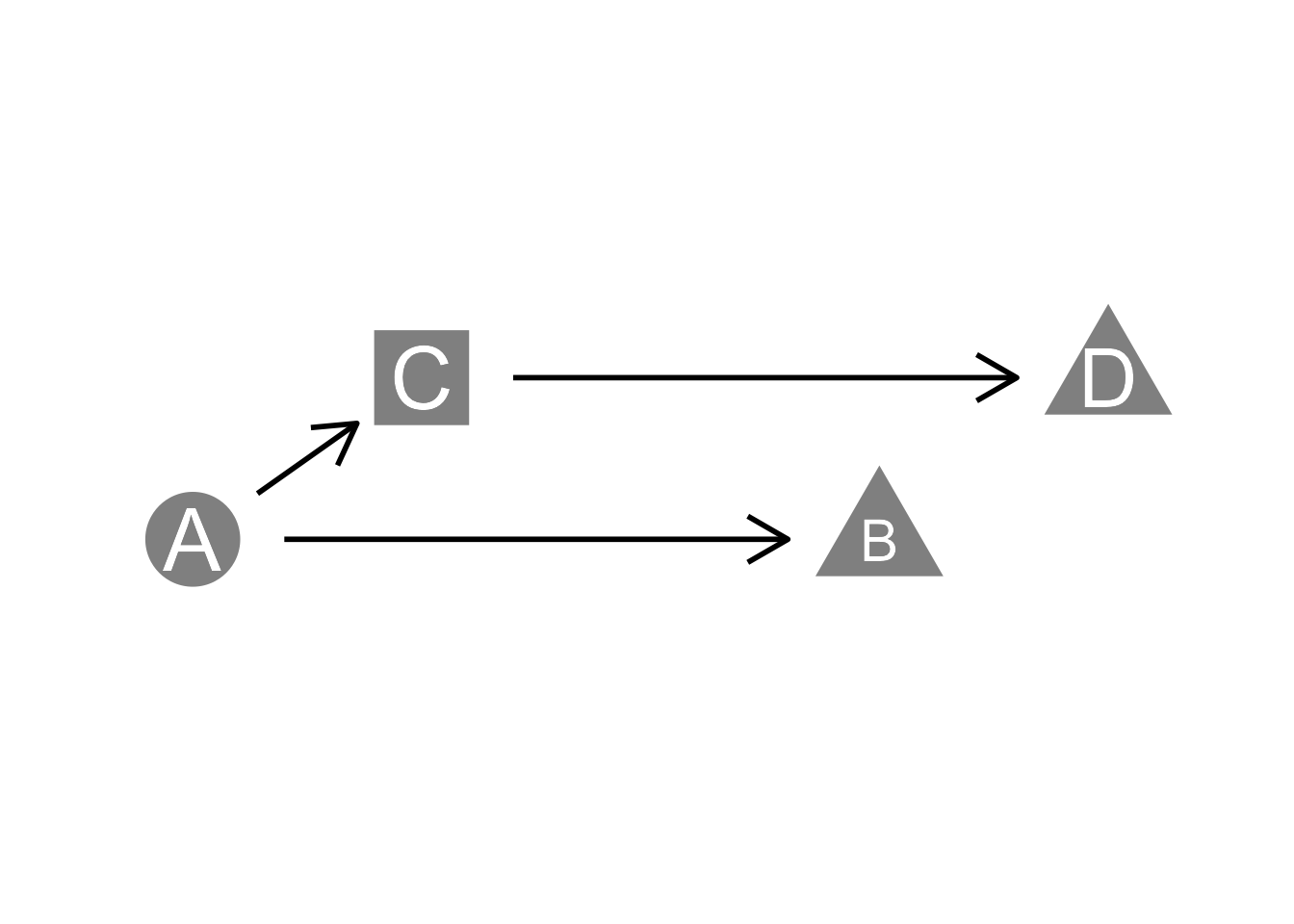1. What are the vertices?
2. Which of these tables shows the edges correctly?
Table 1        Table 2        Table 3
A -> C A -> C A -> C -> D
B -> C A -> B C -> D
A -> B C -> D
1. Explain what’s wrong with each of the other tables.

Problem 14.3: Consider this table of edges:

from to
China Japan
USSR Japan
Germany USA
France Germany
Italy France
Germany UK
Japan UK
USA Japan
USSR Germany
1. How many distinct vertices are there?
2. How many edges are there?